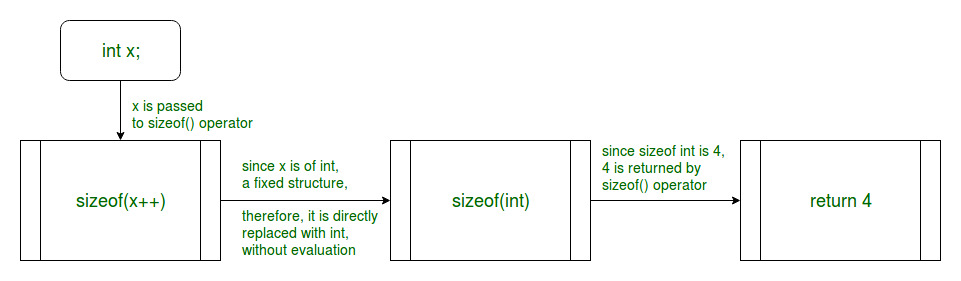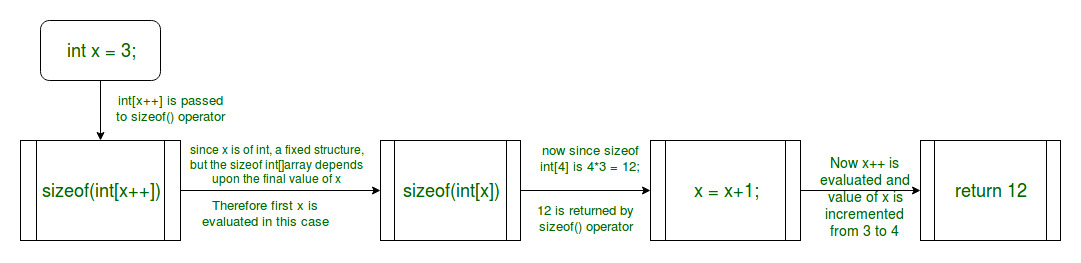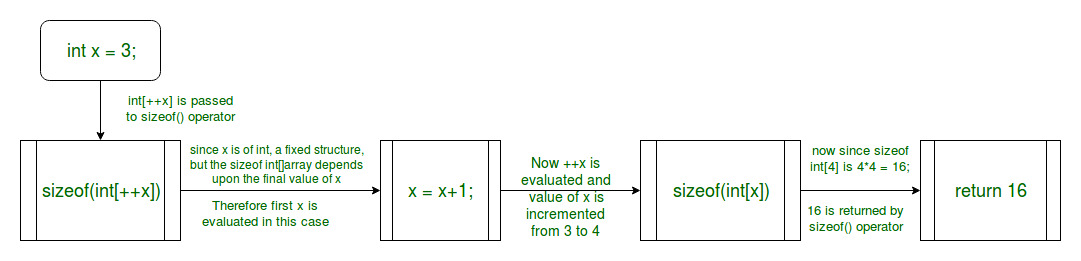# Why does sizeof(x++) not increment x in C?

According to C99 Standards, the sizeof() operator only takes into account the type of the operand, which may be an expression or the name of a type (i.e int, double, float etc) and not the value obtained on evaluating the expression. Hence, the operand inside the sizeof() operator is not evaluated. It is evaluated only if the type of the operand is variable length array because in that case, the size can be determined only after the expression is evaluated.

### Case 1:

When the sizeof() method is passed a fixed size structure:

In this case, the sizeof() operator does not evaluate the parameter. Only the type of the parameter is checked and its size is returned.

Below figure shows the workflow of sizeof() operator in this scenario:Example 1:

The output value of x after increment is still 3 as compared to the expected value which is 4. This is because sizeof operator doesn’t need to evaluate the expression to obtain the size as the data type of the operand doesn’t change and hence the size remains the same.

 `#include ` ` `  `int` `main() ` `{ ` `    ``int` `x = 3; ` `    ``printf``(``"%d\n"``, ``sizeof``(x++)); ` `    ``printf``(``"x = %d"``, x); ` ` `  `    ``return` `0; ` `} `

Output:

```4
x = 3
```

### Case 2:

When the sizeof() method is passed a variable size structure:

In this case, the sizeof() operator evaluates the parameter to see if there is any change of size. If found, then first the size is modified then the final size is returned.

Below example illustrate the sizeof() operator in this scenario:

Example 2:In this example, the sizeof operator needs to evaluate the expression in order to calculate the size of the array which is shown in the figure. Hence, in this case, we get the value of x after incrementation.

 `#include ` ` `  `int` `main() ` `{ ` `    ``int` `x = 3; ` `    ``printf``(``"%d\n"``, ``sizeof``(``int``[x++])); ` `    ``printf``(``"x = %d"``, x); ` ` `  `    ``return` `0; ` `} `

Output:

```12
x = 4
```

Example 3:Again in this example, the sizeof operator needs to evaluate the expression in order to calculate the size of the array which is shown in the figure. In this example, the post-increment operator is used and hence the output in the first line is 16. In the second line, the value of x is printed after incrementation.

 `#include ` ` `  `int` `main() ` `{ ` `    ``int` `x = 3; ` `    ``printf``(``"%d\n"``, ``sizeof``(``int``[++x])); ` `    ``printf``(``"x = %d"``, x); ` ` `  `    ``return` `0; ` `} `

Output:

```16
x = 4
```

Attention reader! Don’t stop learning now. Get hold of all the important DSA concepts with the DSA Self Paced Course at a student-friendly price and become industry ready.

My Personal Notes arrow_drop_upCheck out this Author's contributed articles.

If you like GeeksforGeeks and would like to contribute, you can also write an article using contribute.geeksforgeeks.org or mail your article to contribute@geeksforgeeks.org. See your article appearing on the GeeksforGeeks main page and help other Geeks.

Please Improve this article if you find anything incorrect by clicking on the "Improve Article" button below.

Improved By : rituraj_jain

Article Tags :
Practice Tags :

12

Please write to us at contribute@geeksforgeeks.org to report any issue with the above content.